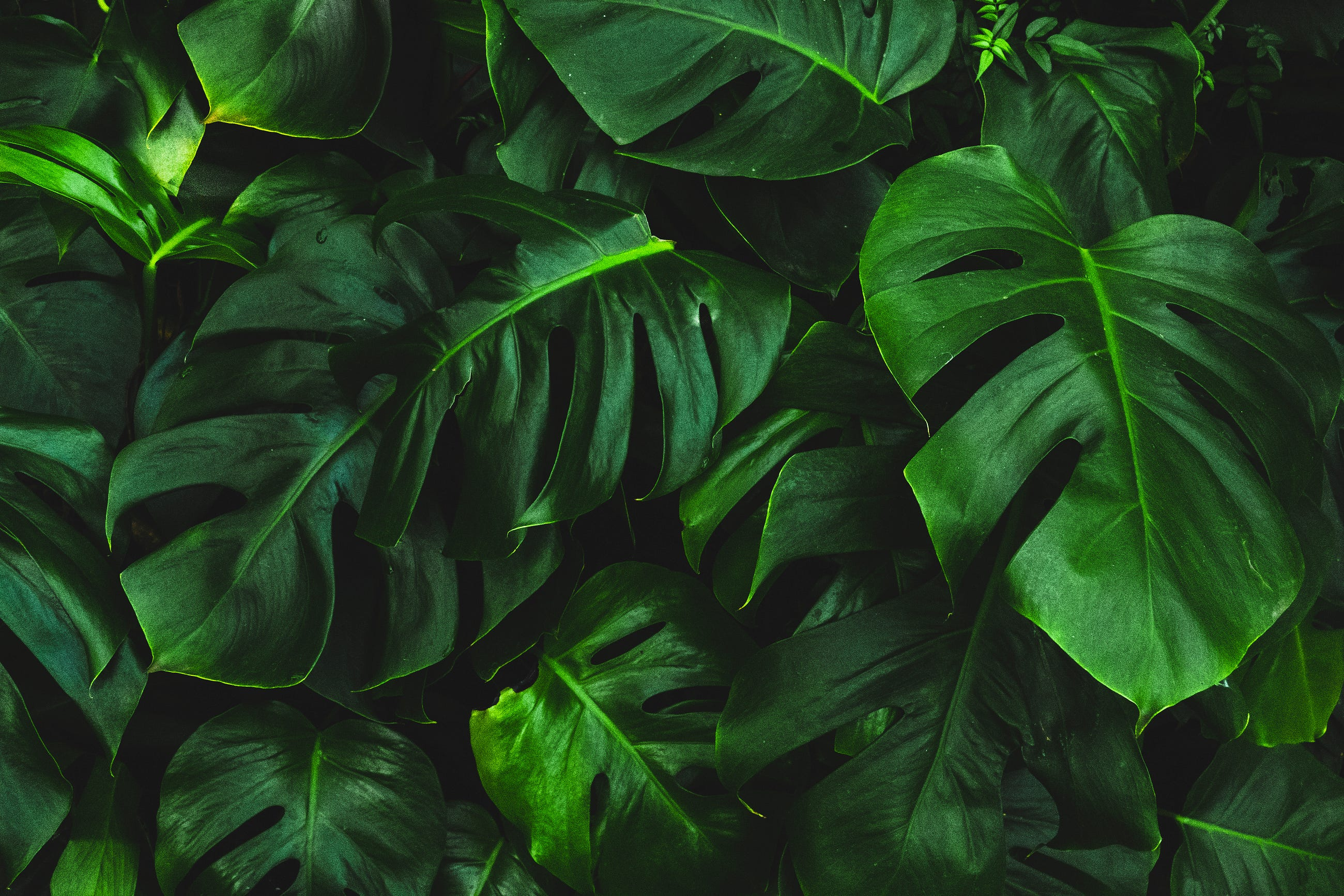# Plant Images Segmentation with Deep Learning

Original article was published by Anis Ismail on Deep Learning on MediumIn the case of the joint model, labels were split between two masks. The first type of mask, corresponding to the first branch, would contain labels 0, 1, 2 with the same significance as above. The second type of masks, corresponding to the second branch, would contain labels 0,1,2 where 2 has the same purpose of label 3 above.

## Training Approach

We have followed the same approach for both model architectures:

• Inputting a 3D RGB image of size 128×128
• Applying preprocessing by normalizing the image colors.
• Augmenting the dataset by changing image orientation horizontally and vertically (Other augmentation techniques such as brightness change distorted the masks so caution must be taken)
• Randomly splitting each dataset between training (90%) and validation (10%). The total number of images from the Sugarbeet dataset was 11,552 and the number of images in the custom dataset was 4,693.
• Using Keras with Tensorflow backend for the entire model including data generators.

## Model Choice

Further research led us to a U-Net model trained on the Sugarbeet dataset (link). Even though the model is only trained for weed detection, we used the weights to transfer the learning to the entire task.

We found other weights for the ResNet 101 model. We believed that ResNet might be a good candidate because it uses residual blocks known to preserve spatial information during the encoding-decoding process. This model was originally trained on ImageNet dataset, so we replaced the last layers with convolutional layers and transferred the learning to our task on the custom dataset.

## Model with Centroid as additional channel

We decided to go with the most straightforward approach by adding an additional channel for the centroid. We will have 4 channels in the output image: Background, Crop, Weed and Centroid. Code for U-Net and ResNet implementations are found in our Github repository.

## Joint model

This model implementation is inspired by the previously mentioned paper by Lottes et Al . The model consists of two outputs and one input (check previous diagram). The first output is the crop/weed mask and the second output is the centroid of the weed plant. Therefore, two types of masks need to be generated to cater for each output branch.

The joint model approach separates the identification of the crops/weeds from centroid detection while still leveraging the learning from the first task to inform the second task. Hence, instead of sequencing the two tasks, it combines them into one by taking advantage of one encoder. Code for U-Net, ResNet, and FC-Densenet joint models are also available on our Github repository.

## Performance Metrics

We relied on the following metrics to test the performance of our models:

• Mean IoU or Intersection over Union, is a common measure for image segmentation. It compute the Intersection over Union for each separate class and then it averaged over the number of classes. The higher the value of the mean IoU, the better.
• We define centroid accuracy as the ratio of number of times a correct centroid is predicted by the model over the actual number of existing centroids.

## Start Training

We trained U-Net model for 360 epochs and ResNet model for 200 epochs with mean IoU and accuracy as training metrics. For roughly the same number of epochs, U-Net outperformed ResNet in IoU and centroid accuracy. The final weights of the models can be found here.

# Results

After training the U-Net model for 360 epochs, the average mean intersection over union (mean IoU) evaluated on 90% of the custom dataset was 0.6 . The centroid accuracy evaluated on 90% of the custom dataset was 0.8 .